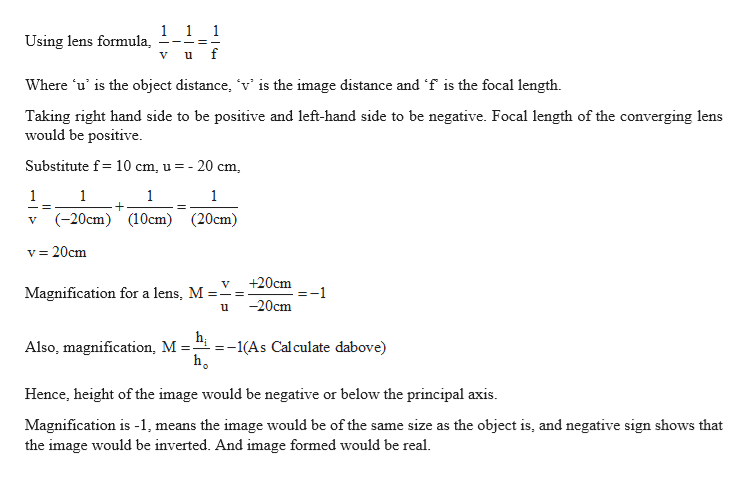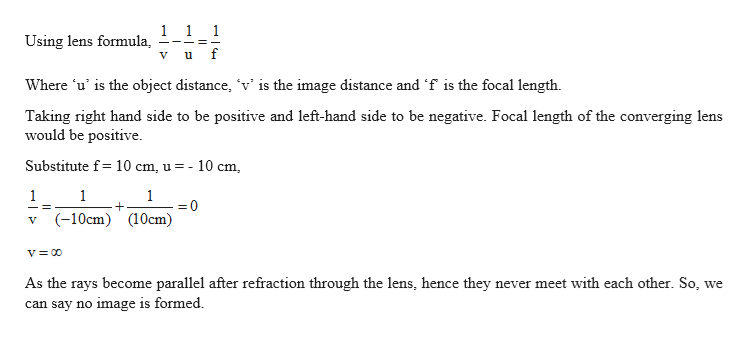# A converging lens has a focal length of 10.0 cm. Locate theimages for object distances of (a) 20.0 cm, (b) 10.0 cm, and(c) 5.00 cm, if they exist. For each case, state whether theimage is real or virtual, upright or inverted, and find themagnification.

Question
22 views

A converging lens has a focal length of 10.0 cm. Locate the
images for object distances of (a) 20.0 cm, (b) 10.0 cm, and
(c) 5.00 cm, if they exist. For each case, state whether the
image is real or virtual, upright or inverted, and find the
magnification.

check_circle

Step 1

Given:

Focal length of the converging lens = 10 cm

Step 2

(a) Calculating the image distance, image type and magnification for object distance 20 cm:help_outlineImage Transcriptionclose1 1 1 Using lens formula, Where 'u' is the object distance, 'v' is the image distance and 'f is the focal length. Taking right hand side to be positive and left-hand side to be negative. Focal length of the converging lens would be positive. Substitute f= 10 cm, u = - 20 cm, (-20cm) (10cm) (20cm) v = 20cm +20cm Magnification for a lens, M =- =-1 -20cm hị =-1(As Calculate dabove) Also, magnification, M =- h. Hence, height of the image would be negative or below the principal axis. Magnification is -1, means the image would be of the same size as the object is, and negative sign shows that the image would be inverted. And image formed would be real. fullscreen
Step 3

(b) Calculating the image distance, image type an...help_outlineImage Transcriptionclose1 1 1 Using lens formula, Where 'u' is the object distance, 'v' is the image distance and 'f is the focal length. Taking right hand side to be positive and left-hand side to be negative. Focal length of the converging lens would be positive. Substitute f= 10 cm, u = - 10 cm, %3D (-10cm) (10cm) As the rays become parallel after refraction through the lens, hence they never meet with each other. So, we can say no image is formed. fullscreen

### Want to see the full answer?

See Solution

#### Want to see this answer and more?

Solutions are written by subject experts who are available 24/7. Questions are typically answered within 1 hour.*

See Solution
*Response times may vary by subject and question.
Tagged in

### Ray Optics